# Preface

A separable equation $$y' = f(x,y)$$ is such differential equation for which the slope function is a product of two functions depending on only one variable: $$f(x,y) = p(x)\,q(y) .$$ Every separable equation can be integrated. Gottfried Leibniz discovered the separable equations in 1691; he also proposed the method for their solutions.

# Separable Equations

A differential equation $$y' = f(x,y)$$ is said to be separable if the slope function is the product of two functions depending on only one variable:  $$f(x,y) = p(x) q(y).$$ Then rewriting the derivative y' in differential form  $$y' = {\text d}y / {\text d}x ,$$ we separate variables:

\begin{equation} \label{EqSeparable.1} \frac{{\text d}y}{{\text d}x} = p(x)\,q(y) \qquad \Longrightarrow \qquad \frac{{\text d}y}{q(y)} = p(x)\, {\text d}x , \qquad q(y) \ne 0 , \end{equation}
each part can be integrated. In other words, a separable differential equation is a differential equation in which the two variables can be placed on opposite sides of the equals sign such that the dx and x terms are on one side and the dy and the y terms are on the other. The dx and dy terms need to be multiplied by the x and y terms, respectively. Note that since we divide by q, we need to exclude from our further consideration the stationary points that are the roots of $$q(y) =0 ;$$ they could be either singular or not. We show how to solve separable differential equations in the following examples.

Example 1: Consider the separable differential equation with polynomial slope:
$y' = x\, y^2 .$
First, we plot its phase portrait:
xy2 = StreamPlot[{1, x*y^2}, {x, -2, 2}, {y, -2, 2}, Frame -> False, Axes -> True, AspectRatio -> 1];
line = Plot[0, {x, -2, 2}, PlotStyle -> {Thick, Green}, PlotLabels -> {"separatrix"}];
Show[line, xy2]

Separating variables, we get
$\frac{{\text d} y}{y^2} = x\,{\text d} x \qquad \Longrightarrow -\frac{1}{y} = \int \frac{{\text d} y}{y^2} = \int x\,{\text d} x = x^2 - C,$
where C is a constant of integration. This gives us the general solution in implicit form:
$2 = y \left( C - x^2 \right).$
As we see from the phase portrait, the trivial solution y ≡ 0 can be obtained by choosing the arbitrary constant to be infinity. It is a separatrix that can be obtained by solving the above differential equation under the initial condition y(k) = 0, where k is any real number. We can extract a particular solution by setting C some numerical value, for example, C = 1, which is the same as to set up the initial condition y(0) = 2.
 Plot[2/(1 - x^2), {x, -2, 2}, PlotStyle -> {Thick, Red}]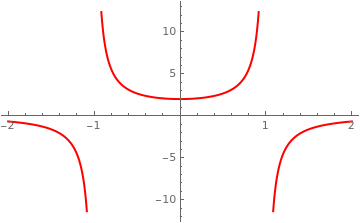Points (±1,0) are not singular points because at this points the solution can be obtained from the general solution by setting the arbitrary constant C = ∞, and its streamline has infinite slope at these points. Therefore, the equilibrium solution y ≡ 0 is not a singular solution and the general solution contains all possible solutions upon choose an appropriate value of a constant C.    ■
Example 2: Consider another separable equation
$y' = x\, y^{1/2} , \qqyad y > 0.$
First, we plot its phase portrait:
 xsqrt = StreamPlot[{1, x*Sqrt[y]}, {x, -2, 2}, {y, -0.3, 2}, Frame -> False, Axes -> True, AspectRatio -> 1] line = Plot[0, {x, -2, 2}, PlotStyle -> {Thick, Green}, PlotLabels -> {"separatrix"}]; Show[line, xsqrt]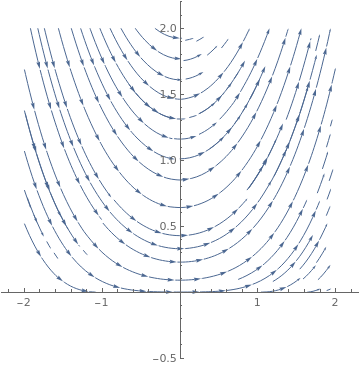Separation of variables yields the general solution
$\frac{{\text d}y}{\sqrt{y}} = x\,{\text d}x \qquad\Longrightarrow \qquad \sqrt{y} = \left( \frac{x^2}{4} + C \right) .$
Then we plot some members of the general solution along with the separatrix y ≡ 0.
 f[a_, x_] := (a + x^2 /4)^2; parameters = {0, 0.5, 1, 1.5, 2}; Plot[Evaluate[f[#, x] & /@ parameters], {x, -3, 3}, PlotRange -> {0, 10}, PlotStyle -> Thick, PlotLegends -> Table[Row[{"C=", j}], {j, parameters}]]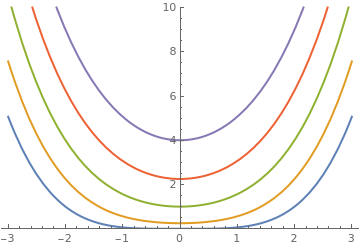The trivial solution y ≡ 0 cannot be obtained from the general solution for any value of constant C, including infinity. Therefore, it is a singular solution. This function touches one solution $$y = x^4 /16$$ that corresponds to C = 0.    ■
Example 3: The general solution to the equation $$y ' = x^2/y^2 /\sqrt{4-x^2}$$ can be found by separation of variables:
$y^2 {\text d}y = \frac{x^2 {\text d}x}{\sqrt{4-x^2}} \qquad\Longrightarrow \qquad \frac{y^3}{3} = \int y^2 {\text d}y = \int \frac{x^2 {\text d}x}{\sqrt{4-x^2}} = -\frac{x}{2} \,\sqrt{4-x^2} + 2\,\arcsin \left( \frac{x}{2} \right)$
because
Integrate[x^2/Sqrt[4 - x^2], x]
-(1/2) x Sqrt[4 - x^2] + 2 ArcSin[x/2]
So we obtain its general solution in implicit form:
$y^3 = 6\,\arcsin \left( \frac{x}{2} \right) - \frac{3x}{2} \,\sqrt{4-x^2} +C,$
where C is a constant of integration. Mathematica can find its solution directly:
DSolve[y'[x] == x^2 /y^2 /Sqrt[4-x^2], y, x] // Flatten
{ y -> Function[{x}, -(-3/2)^(1/3) (-x Sqrt[4-x^2] + 4 ArcSin[x/2] + 2 C)^(1/3)],
y -> Function[{x}, (3/2)^(1/3) (-x Sqrt[4-x^2] + 4 ArcSin[x/2] + 2 C)^(1/3)],
y -> Function[{x}, (-1)^(2/3) (3/2)^(1/3) (-x Sqrt[4-x^2] + 4 ArcSin[x/2] + 2 C)^(1/3)]}
By plotting the corresponding phase portrait, we see that the origin is a critical point:
StreamPlot[{1, x^2/y^2/Sqrt[4 - x^2]}, {x, -2.1, 2.1}, {y, -2.1, 2.1}, Frame -> False, Axes -> True, AspectRatio -> 1]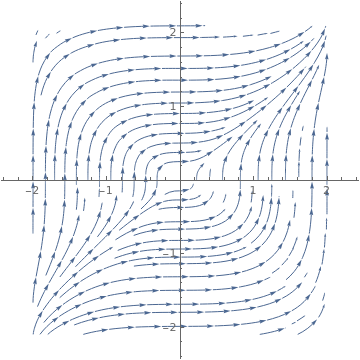The vertical lines x = ±2 are fences for solutions because they do not exist outside the interval |x| ≤2.    ■
Example 4: Consider the separable differential equation:   $$y'= xy/(1+x^2 ).$$ First, we separate variables and integrate
$\frac{{\text d}y}{y} = \frac{{\text d}x\,x}{1+ x^2} \qquad \Longrightarrow \qquad \ln Cy = \frac{1}{2} \, \ln (1+x^2 ) = \ln \sqrt{1+ x^2} .$
Therefore, the general solution (which is a family of functions containing arbitrary constant C) is
$C\,y^2 = 1 + x^2 .$

DSolve[{y'[x] == x*y[x]/(x^2 + 1)}, y[x], x]
Plot[{Sqrt[1 + x*x], 4*Sqrt[1+x*x], 0.4*Sqrt[1+x*x], 8*Sqrt[1+x*x]}, {x, 0, 5}, PlotLabel -> "Solutions to Example 1.3.1 "]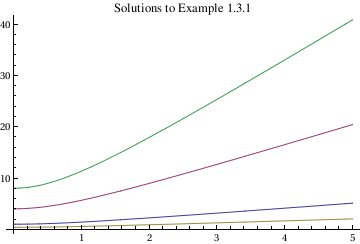In this command sequence, the first line of code asks Mathematica to find the solution to this separable differential equation.
The second line contains commands to plot different possible solutions to this separable differential equation based on the value of the integration constants. None of the subcommands of Plot are new in this command sequence and they have already been explained earlier.

We would like to use advantage of knowing Mathematica to solve the equation by direct integration and then to plot those solutions:

Clear[t,y];
t0 = 0; y0 = 2; f[t_] = t/(1 + t^2); g[y_] = 1/y;          (* define the initial values and the slope functions *)

F[t_] = Integrate[f[t], t]; G[y_] = Integrate[g[y], y];
gensol = Solve[G[y] == F[t] + c, y];
const = Solve[y == y0 /. gensol /. t -> t0, c];
sol[t_] = y /. gensol /. const[]                                (* solution of the IVP *)
Out = {2 Sqrt[1 + t^2]}
Plot[sol[t], {t, -2, 2}]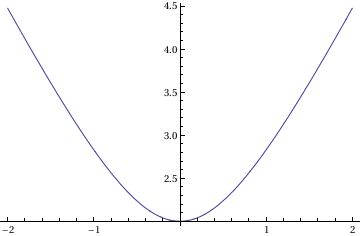In this syntax, the first command, Clear, clears out any previously existing values for the two variables, allowing you to redefine them.
The second command defines the different initial conditions and the two functions that you are trying to solve.
The first command line tells Mathematica which functions you want to integrate.
The fourth and fifth lines of codes tell Mathematica how you want to define the general solution and how you want to solve for the integration constant c.
The sixth line gives the final solution to this separable differential equation (this is also an initial value problem).
The final line tells Mathematica which function to plot and the range.

Finally, we plot the corresponding phase portrait with separatrix y ≡ 0.

line = Plot[0, {x, -2.2, 2.2}, PlotStyle -> {Thick, Green}, PlotLabels -> {"separatrix"}]
phase = StreamPlot[{1, x*y/Sqrt[1 + x^2]}, {x, -2.1, 2.1}, {y, -2.1, 2.1}, Frame -> False, Axes -> True, AspectRatio -> 1]
Show[line, phase]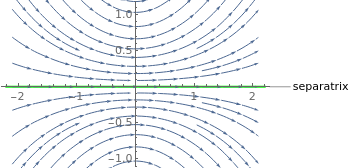■

In Mathematica, as the previous example shows, you can insert comments by adding a comment surrounded by stars as in (* solution of the IVP *) and then parentheses. Even when variables can be separated, the final solution might be accompanied by a warning message from Solve, or it might only be given as an InverseFunction object.

Example 5: Consider a separable differential equation:    y' +ycos(x) =0
sol = DSolve[y'[x] == -Cos[x]*y[x], y[x], x]
Out= {{y[x] -> E^-Sin[x] C}}
toplot = Table[E^-Sin[x] C /. C -> i, {i, -5, 5}];
Plot[Evaluate[toplot], {x, 0, 4*Pi}]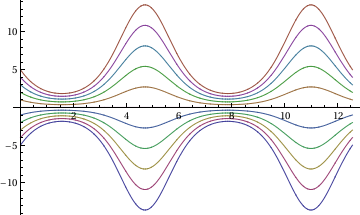■
Example 6: Consider a separable differential equation:    x(y+1)(y-3) dx +(1+5y)(x+2) dy =0. We solve this equation by separating variables and then integrating:

Integrate[x/(x + 2), x] + Integrate[(1 + 5*y)/(y + 1)/(y - 3), y] == c
Out = x - 2 Log[2 + x] + 4 Log[-3 + y] + Log[1 + y] == c
solution = E^x *(x + 2)^(-2) * (y - 3)^4 *(1 + y)
Out = (E^x (-3 + y)^4 (1 + y))/(2 + x)^2
factored = Factor[Dt[solution]] /. {Dt[x] -> dx, Dt[y] -> dy}
Out = (E^x (-3 + y)^3 (2 dy - 3 dx x + dy x + 10 dy y - 2 dx x y + 5 dy x y + dx x y^2))/(2 + x)^3
diffExpr = Collect[factored[], {dx, dy}]
Out = dy (2 + x + 10 y + 5 x y) + dx (-3 x - 2 x y + x y^2)
dxPart = Factor[Coefficient[diffExpr, dx]]
Out = x (-3 + y) (1 + y)
dyPart = Factor[Coefficient[diffExpr, dy]]
Out = (2 + x) (1 + 5 y)
dxPart*dx + dyPart*dy == 0
Out = dx x (-3 + y) (1 + y) + dy (2 + x) (1 + 5 y) == 0
The given differential equation has two equilibrium solutions y = 3 and y = -1. Next, we plot the phase portrait.
Integrate[x/(x + 2), x] + Integrate[(1 + phase = StreamPlot[{1, -x*(y + 1)*(y - 3)/(1 + 5 y)/(x + 2)}, {x, -4, 4}, {y, -4, 4}, Frame -> False, Axes -> True, AspectRatio -> 1]
line1 = Plot[3, {x, -4, 4}, PlotStyle -> {Thick, Green}, PlotLabels -> {"equilibrium"}]
line2 = Plot[-1, {x, -4, 4}, PlotStyle -> {Thick, Green}, PlotLabels -> {"equilibrium"}]
Show[line1, line2, phase, PlotRange -> {-4, 4}]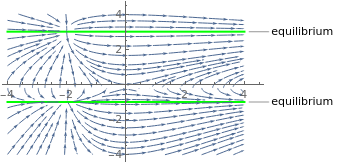■
Example 7: (Newton's Law of Cooling) Separable equations arise in a wide range of application problems. The time of death of a murder victim is an important question on many popular movies and television programs. How does a forensic scientist or a medical examiner determine the time of death? Human beings have a temperature of 98.6F or 36.6C. If the surrounding temperature is cooler, then the body will cool down after death. Eventually, the temperature of the body will match the temperature of the environment. We should not expect the body to cool at a constant rate either. Think of how a hot cup of coffee or tea cools. The liquid will cool quite quickly during the first few minutes but will remain relatively warm for quite a long period.

The answer to our forensic question can be found by using Newton's law of cooling, which tells us that the rate of change of the temperature of a object is proportional to the difference between the temperature of the object and the temperature of the surrounding medium. Newton's law of cooling can be easily stated as a differential equation,

$\frac{{\text d}T}{{\text d}t} = k \left( T- T_m \right) ,$
where T is the temperature of the object, Tm is the temperature of the surrounding medium, and k is the proportionality constant.

Suppose that the temperature of the surrounding environment is 21C, and we know from experience that a body under these conditions cools off approximately 1C during the first hour after death. In order to determine a formula for the time of death, we must solve the initial value problem

$\frac{{\text d}T}{{\text d}t} = k \left( T- 21 \right) , \qquad T(0)= 36.6 ,$
where T(1) = 35.6. If we rewrite the equation as
$\frac{{\text d}T}{T-21} = k \, {\text d}t ,$
we see that this equation is separable. Integrating both sides of the last equation, we obtain $$\ln \left( T-21 \right) = kt+C ,$$ since we are assuming that T > 21. Therefore,
$T-21 = e^{kt +C} = e^C \, e^{kt} = K\, e^{kt} ,$
where $$K= e^C$$ is an arbitrary constant. The initial condition T(0) = 36.6 tells us that K = 15.6. So
$T (t) = 15.6\, e^{kt} +21.$
Since we know the value of the temperature after 1 minute to be T(1) = 35.6, we get the equation to determine the coefficient k:
$T (1) = 35.6 = 15.6\, e^{k} +21 \qquad \Longrightarrow \qquad e^k = 14.6/15.6 \approx 0.935897 .$
Taking logarithm, we get
$k = \ln \frac{14.6}{15.6} \approx -0.0662494 \qquad \Longrightarrow \qquad T(t) = 21 + 15.6 \,e^{-0.0662494 \, t} .$
■
Example 8: A ball at the temperature 1200K (in kelvin units) is allowed to cool down in air at an ambient temperature of 300K. Assuming heat is lost only due to radiation, we can apply the Stefan–Boltzmann law. Then the differential equation for the temperature of the ball is given by
$\frac{{\text d}\theta}{{\text d}t} = - 2.2067\times 10^{-12} \left( \theta^4 - 81\times 10^8 \right) , \qquad \theta (0) =1200 .$
We first separate variables
$\frac{{\text d}\theta}{ \theta^4 - 81\times 10^8} = - 2.2067\times 10^{-12}{\text d} t \qquad \Longrightarrow \qquad \int \frac{{\text d}\theta}{ \theta^4 - 81\times 10^8} = - 2.2067\times 10^{-12} t + C .$
The integral at the left side we dedicate to Mathematica:
Integrate[1/(v^4 - b), v]
-((2 ArcTan[v/b^(1/4)] - Log[b^(1/4) - v] + Log[b^(1/4) + v])/( 4 b^(3/4)))
Therefore, we get the general solution in implicit form:
$\ln \left\vert 300-\theta \right\vert - \ln \left[ 300+\theta \right] -2\,\arctan \frac{\theta}{300} = -4\times 300^3 \left( 2.2067\times 10^{-12} t + C \right) .$
To satisfy the initial condition, we set θ = 1200 and t = 0 to obtain the condition for C:
$\ln \frac{900}{1500} - 2\,\arctan (4) = -4\times 300^3 C ,$
so
$C = \frac{1}{108,000,000} \left[ \ln \left( \frac{5}{3} \right) + 2\,\arctan (4) \right] \approx 2.9282 \times 10^{-8} .$
■
Example 9: (Mixing Problems) There is a large class of problems in modeling known as mixing problems. These problems refer to situations where two are more substances are mixed together in a container or containers. For example, we might wish to model how chemicals are mixed together in a refinery, how pollutants are mixed together in a pond or a lake, how ingredients are mixed together when brewing beer, or even how various greenhouse gases mix together across different layers of the atmosphere.

Suppose that we have a large tank containing 4000 liters of water and that water containing 0.01 kg of salt per liter flows into the tank at a rate of 4 liters per minute. If the tank is also draining at a rate of 4 liters per minute, the water level in the tank will remain constant. We will assume that the water in the tank is constantly stirred so that the mixture of salt and water is uniform in the tank.

We can model the amount of salt in the tank using differential equations. If x(t) is the amount of salt in the tank at time t, then the rate at which the salt is changing in the tank is the difference between the rate at which salt is flowing into the tank and the rate at which it is leaving the tank, or

$\frac{{\text d}x}{{\text d}t} = \mbox{rate in} - \mbox{rate out} .$
Of course, the salt flows into the tank at the rate of 4x0.01 = 0.04 kg (or 40 grams) of salt per minute. However, the rate at which the salt leaves the tank depends on x(t), the amount salt in the tank at time t. At time t, there is x(t)/4000 kg of salt in one liter. Therefore, salt flows out of the tank at a rate of 4 x(t)/4000 = x(t)/1000 kg per minute. The differential equation now becomes
$\frac{{\text d}x}{{\text d}t} = 0.04 - \frac{x}{1000} , \qquad x(0) =0.$
This equation is separable,
$\frac{{\text d}x}{40-x} = \frac{{\text d}t}{1000} .$
Integrating both sides of the equation, we have
$- \ln |40-x| = \frac{t}{1000} +k .$
Consequently,
$40-x(t) = C\, e^{-0.0001\,t} ,$
where $$C= e^{-0.0001\,k} .$$ From our initial condition, we can quickly determine that C = 40 and
$x(t) = 40 - 40\, e^{-0.0001\,t}$
models the amount of salt in the tank at time t.    ■

Theorem: Let f: Ω ⊂ ℝ² → ℝ. Then f(x,y) = p(x) q(y) for all (x,y) ∈ Ω if and only if

$f(a,b)\, f(x,y) = f(x,b)\,f(a,y) \qquad \mbox{for all} \quad (a,b), \ (x,y) \in \Omega.$

Corollary: Let f: Ω ⊂ ℝ² → ℝ and assume that there exists $$( x_0 , y_0 ) \in \Omega$$ such that $$f(x_0 , y_0 ) \ne 0 .$$ Then f(x,y) = p(x) q(y) for all (x,y) ∈ Ω if and only if

$f(x,y) = \frac{f(x, y_0 )\, f(x_0 , y)}{f( x_0 , y_0 )} \qquad \mbox{for all} \quad (x,y) \in \Omega.$
Moreover, if the above condition holds, we can choose $$\displaystyle p(x) = \frac{f(x, y_0 )}{f(x_0 , y_0 )}$$ and $$\displaystyle q(y) = f(x_0 , y)$$ as factors of f(x,y).
Example 10: We consider the function
$f(x,y) = e^{x^2 + 4y^2} \left( \cos (2x+y) + \cos (2x-y) \right) .$
If we choose $$(x_0 , y_0 ) = (0,0)$$ for which $$f(0,0) = 2 ,$$ then
$p(x) = \frac{f(x,0)}{f(0,0)} = e^{x^2} \cos 2x \qquad\mbox{and} \qquad q(y) = f(0,y) = 2\,e^{4y^2} \cos 2y .$
Now it is an easy calculation with the trigonometric identities to verify that indeed $$f(x,y) = p(x)\, q(y) .$$       ▪
Example 11: Here is a single ODE for tumor growth model:
$\frac{{\text d} V}{{\text d} t} = \lambda\,e^{-\alpha \,t} V(t) , \qquad V(0) = V_0 ,$
where V is tumor volume, t is time, and λ, α are constants. Separation of variables yields
$\frac{{\text d} V}{V} = \lambda\,e^{-\alpha \,t} {\text d}t \qquad \Longrightarrow \qquad \ln V = C - \frac{\lambda}{\alpha}\, e^{-\alpha \,t} ,$
where C is a constant of integration. Raising to exponent, we get the explicit solution
$V(t) = V_0 \exp \left\{ - \frac{\lambda}{\alpha} \left( e^{-\alpha \,t} -1 \right) \right\} .$
The tumor growth equation has one unstable equilibrium solution V ≡ 0.       ▪

1. Cid, J.A., A simple method to find out when an ordinary differential equation is separable, International Journal of Mathematical Education in Science and Technology, 2009, Vol. 40, No. 5, pp. 659--662. https://doi.org/10.1080/00207390802136578
2. Plaat, O., Separation of variables, The American Mathematical Monthly, 1968, Vol. 75, No. 8, pp. 844--847. doi: 10.2307/2314332
3. Scott, D., When is an ordinary differential equation separable?, The American Mathematical Monthly, 1985, Vol. 92, No. 6, pp. 422--423. doi: 10.2307/2322452
4. Viazminsky, C.P., On separation of variables, Department of Physics, University of Aleppo, Syria.